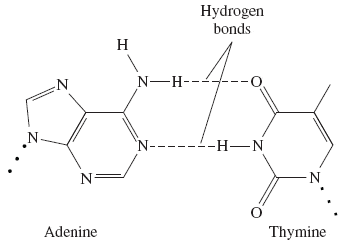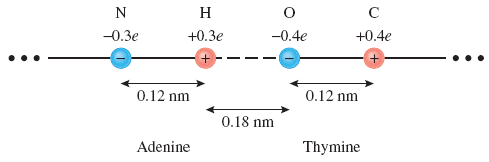# Hydrogen bonding force problem

• StormPix
In summary, the base pair adenine and thymine in a DNA molecule is held together by two hydrogen bonds. Using point charges and the given information, the magnitude of the net electric force along the hydrogen bond was calculated to be 3.99x10^-10 N, with two repelling forces between N&O and H&C and two attractive forces between N&C and H&O. The values for N, H, O, and C were corrected to account for the charge of an electron.

## Homework Statement

In a DNA molecule, the base pair adenine and thymine is held together by two hydrogen bonds (see figure below).Let's model one of these hydrogen bonds as four point charges arranged along a straight line. Using the information in the figure below, calculate the magnitude of the net electric force along this hydrogen bond.## Homework Equations

F=(k*|q1|*|q2|)/r^2

## The Attempt at a Solution

Since we are looking at 4 point charges, we need 4 equations. They are:

k = 8.99x10^9 (N*m^2)/C^2
N = -3x10^-10 C
O = -4x10^-10 C
H = 3x10^-10 C
C = 4x10^-10 C

F_no = (k*|N|*|O|) / ((3x10^-10 m)^2) = -1.20x10^10 N -> negative as repelling force
F_nc = (k*|N|*|C|) / ((4.2x10^-10 m)^2) = 6.12x10^9 N
F_ho = (k*|H|*|O|) / ((1.8x10^-10 m)^2) = 3.33x10^10 N
F_hc = (k*|H|*|C|) / ((3x10^-10 m)^2) = -1.2x10^10 N -> negative as repelling force

Since we are asked for find the magnitude of the net electric force, we need to sum all 4 forces together.

ΣF = F_no + F_nc + F_ho + F_hc = 1.54x10^10 N

... Opps. So I must have done something wrong.

The hint given was "You need 4 eqns: pair N&O, N&C, H&O, H&C and use the charges & distances appropriate for each pair to get a net magnitude between the bases." ... which to my knowledge I have here.

So not sure what I did wrong. Any help would be much appreciated.
[/B]

StormPix said:
N = -3x10^-10 C
O = -4x10^-10 C
H = 3x10^-10 C
C = 4x10^-10 C

Check these numbers. What does "e" stand for in the picture?

It would be clearer to leave out k and the unit charge of an electron as common factors to bring in later. E.g. for N::O write -0.3*0.4/(0.12+0.18)2.

TSny said:
Check these numbers. What does "e" stand for in the picture?

Ah! Small but critical oversight on my part. "e" meant electron ... so that each base pair is a factor .3 or .4 charge of an electron.

This would make:

N = (-.3)(1.602x10^-19 C) = -4.806x10^-20 C
H = (.3)(1.602x10^-19 C) = 4.806x10^-20 C
O = (-.4)(1.602x10^-19 C) = -6.408x10^-20 C
C = (.4)(1.602x10^-19 C) = 6.408x10^-20 C

Using these new values for N, H, O, C I get

F_no = -3.08x10^-10 N -> repelling force
F_nc = 1.57x10^-10 N
F_ho = 8.55x10^-10 N
F_hc = -3.08x10^-10 N -> repelling force

Such that,

ΣF = 2*(-3.08x10^-10 N) + 1.57x10^-10 N + 8.55x10^-10 N = 3.99x10^-10 N which ended up be the correct answer.

Thanks a lot.